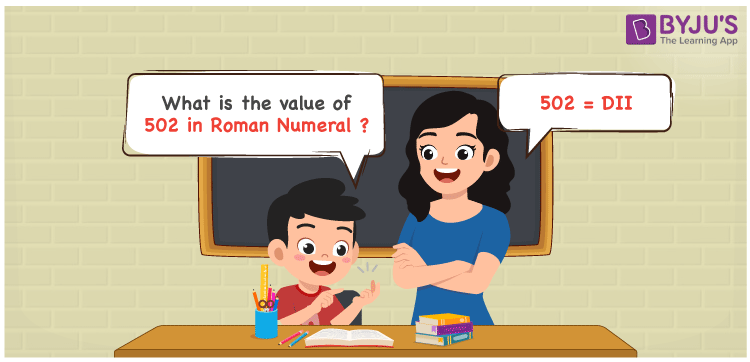# 502 in Roman Numerals

DII is 502 in Roman numerals. To convert 502 to Roman Numerals, write it in expanded form, i.e. 502 = 500 + 1 + 1, then replace the changed numbers with corresponding Roman numerals to get 502 = D + I + I = DII. In this article, we’ll show you how to convert 502 to Roman numerals accurately.

 Number Roman Numeral 502 DII## How to Write 502 in Roman Numerals?

In order to write 502 in Roman Numerals, it has to be written in expanded form, i.e.

502 = 500 + 2

502 = D + II

502 = DII

## Video Lesson on Roman Numerals## Frequently Asked Questions on 502 in Roman Numerals

Q1

### Write 502 in Roman Numerals.

In Roman Numerals, 502 is written as DII.
Q2

### Why is 502 in Roman Numerals Written as DII?

We know that in roman numerals, we write 2 as II, 500 as D, and 100 as C. Therefore, 502 in roman numerals is written as 502 = 500 + 2 = D + II = DII.
Q3

### How to convert 502 into Roman Numerals?

The conversion of 502 into Roman Numerals includes breaking the number according to its place value.
Hundreds = 500 = D
Ones = 2 = II
Number = 500 + 2 = D + II = DII